### Generalized Coefficient of Determination

Cox and Snell (1989, pp. 208–209) propose the following generalization of the coefficient of determination to a more general linear model: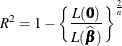where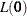is the likelihood of the intercept-only model,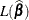is the likelihood of the specified model,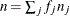is the sample size,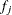is the frequency of the jth observation, and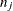is the number of trials when events/trials syntax is specified or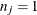with single-trial syntax.

The quantity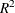achieves a maximum of less than one for discrete models, where the maximum is given by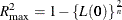Nagelkerke (1991) proposes the following adjusted coefficient, which can achieve a maximum value of one: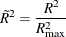Specifying the NORMALIZE option in the WEIGHT statement makes these coefficients invariant to the scale of the weights.

Like the AIC and SC statistics described in the section Model Fitting Information,and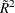are most useful for comparing competing models that are not necessarily nested—larger values indicate better models. More properties and interpretation ofandare provided in Nagelkerke (1991). In the Testing Global Null Hypothesis: BETA=0 table,is labeled as RSquare andis labeled as Max-rescaled RSquare.   Use the RSQUARE option to requestand.